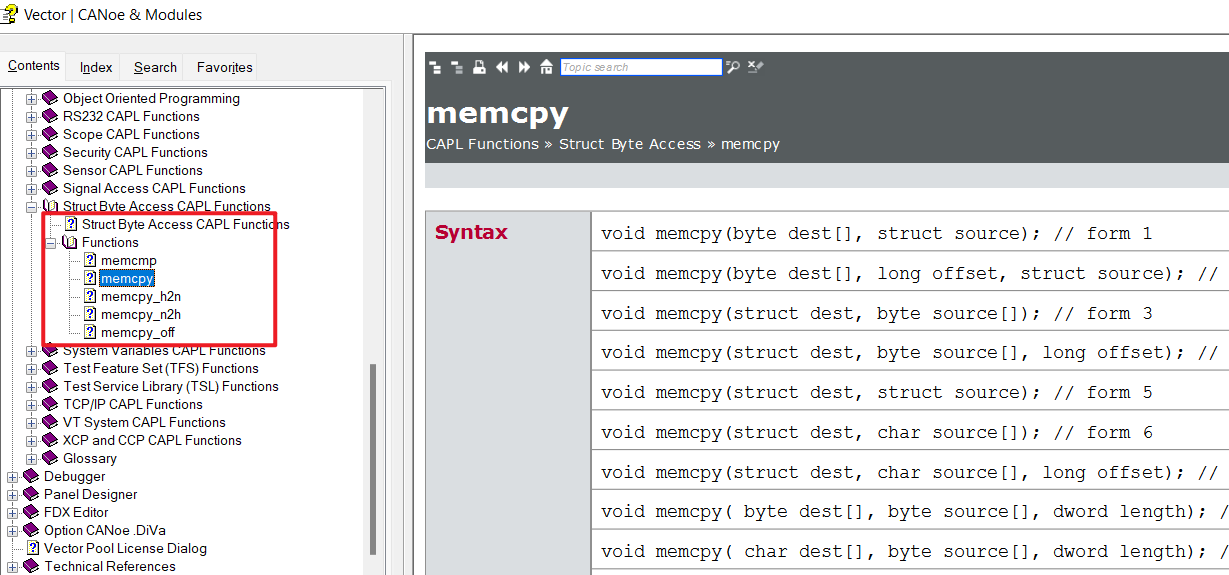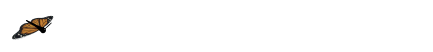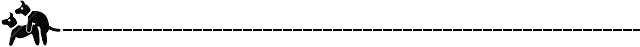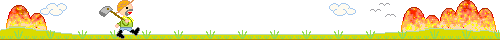IP地址

# 📙 问题1：怎么将byte A={0x01,0x02,0x03,0x04,0x05}赋值byte B？

Python入门

``````On key 'b'
{
long i;
byte A={0x01,0x02,0x03,0x04,0x05};
byte B;
//怎么将A数组赋值给B数组？
for(i=0;i< elCount(A);i++)
{
B[i] = A[i];
}
}

``````
• 内置memcpy函数做法：
• void memcpy( byte dest[], byte source[], dword length); // form 8
``````On key 'h'
{
byte A={0x01,0x02,0x03,0x04,0x05};
byte B;
memcpy(B,A,elCount(A));
write("**0x%x,0x%x,0x%x,0x%x,0x%x",B,B,B,B,B);
}
``````

# 📙 问题2：怎么把 byte A={49,50,51,52,53} 赋值给 char B?

SPL

• 一般做法，就是循环赋值，如下：
``````On key 'j'
{
long i;
byte A={49,50,51,52,53};
char B;

for(i=0;i< elCount(A);i++)
{
B[i] = A[i];
}
write("**%s",B);
}
//输出：**12345

``````
• 内置memcpy函数做法：
• void memcpy( char dest[], byte source[], dword length); // form 9
``````On key 'k'
{
long i;
byte A={49,50,51,52,53};
char B;
memcpy(B,A,elCount(A));

write("**%s",B);
}
//输出：**12345
``````

# 📙 问题3：和问题2 时互操作，将 char A="12345"转为 byte B

• 内置memcpy函数做法：
• void memcpy( byte dest[], char source[], dword length); // form 10
``````On key 'l'
{
char A="12345";
byte B;
memcpy(B,A,strlen(A));

write("**%d,%d,%d,%d,%d",B,B,B,B,B);
}

``````

# 📙 问题4：字符串赋值操作，将 char A="12345"赋值给 char B

ios上架苹果商店流程

• void memcpy( byte dest[], char source[], dword length); // form 10
``````On key 'm'
{
char A="12345";
char B;
memcpy(B,A,strlen(A)); //方法1：
//strncpy (B,A,elcount(A)); //方法2：
//snprintf(B, elcount(B), "%s",A);//方法3
write("**%s",B);
}

``````

# 📙 问题5：怎么比较是否相等两个数组byte A和byte B 是否相等呢

• 一般做法，可能就是在for循环中一一对比，现在可以用memcmp 方法
• int memcmp(byte buffer1[], byte buffer2[], dword size); // form 4
• 返回值为 0，则两者相等
``````On key 'n'
{
long Result;
byte A={0x01,0x02,0x03,0x04,0x05};
byte B={0x01,0x02,0x03,0x04,0x06};
Result = memcmp(A, B ,elCount(A));
write("byte A and byte B equal? %d",Result);
}
// 结果：byte A and byte B equal? -1
``````

# 📙 问题6：两个char [] 数组（字符串）比较可以用 strncmp函数

• long strncmp(char s1[], char s2[], long len);
• 返回值为 0，则两者相等
``````On key 'v'
{
char s1 = "Vector";
char s2 = "Vector";

if(strncmp(s1,s2,strlen(s1)))
write("not equal");
else
write("equal");
}
``````

# 📙 总结

memcpy 和memcmp 函数 根据参数不同重载了很多，不管是byte 数组，还是char 字符串，还是struct 结构体，都是在内存中占用连续内存的，都可以使用memcpy相互赋值。AndroidStudioITredis分片集群添加新节点exception

• 🚩要有最朴素的生活，最遥远的梦想，即使明天天寒地冻，路遥马亡！
• 🚩如果这篇博客对你有帮助，请 “点赞” “评论”“收藏”一键三连 哦！码字不易，大家的支持就是我坚持下去的动力。